# Feature Tracking and Optical Navigation

This article is a simplified version of the research report that aims at identifying and tracking craters in images for optical navigation in space. We first survey at existing image processing techniques. We then proceed to bootstrapping a deep neural network classifier with the help of TensorFlow Object Detection API and images from NASA’s Detecting Crater Impact Challenge. We then implement a preliminary tracking algorithm that stores images and computes mean squared error to detect if the crater has already been seen before.

In this article, we additionally go into more details of the tracking algorithm. For the code, check the object-detection branch of our GitHub repository.

## Background

Ever since humans landed on the moon, it became clear than deep space travel is a possibility in the future. One of the biggest issues faced by satellites and probes that we have sent into space is that they do are unable to react to the presence of other astronomical objects real time. This means that they must rely on scientists back at Earth for navigation. Satellite images get sent back to Earth for scientists to study the situation. At the least, the time delay slows down the mission progress and causes overhead.

In this article, we attempt to provide a method to track craters on astronomical objects. We will first identify potential craters on the astronomical object. Then we will start tracking these potential crates and calculate how far these craters have been displaced since the last image was taken. We can then feed these inputs into a navigation filter for thr actual navigation.

## Our Workflow

This research area is very broad and involves three big topics – identification of craters, tracking of craters, and navigation. This article will mainly focus on the crater identification and will briefly touch upon tracking.

Our workflow, for tackling the identification of features, is to design two different models that achieve the same results simultaneously. The first will use pure image processing techniques to identify craters. The second will be a mixture of image processing and machine learning techniques. Then we will either choose one of either models, or a combination of both, based on their robustness.

For the second question, tracking craters, we discuss a preliminary algorithm we designed.

## Image Processing

We used off-the-shelf feature detection algorithms to test their robustness. OpenCV offers many feature detectors like Hough Circle Finding method and Harris Corner Detection. These feature detectors provided by OpenCV allow for decent crater findings, but need significant tuning. This leads to questions regarding robustness and automation, and this is where machine learning might lead to better results.

Here is a sample result we got after a lot of fine tuning. As you can see, the results are decent, but not very precise.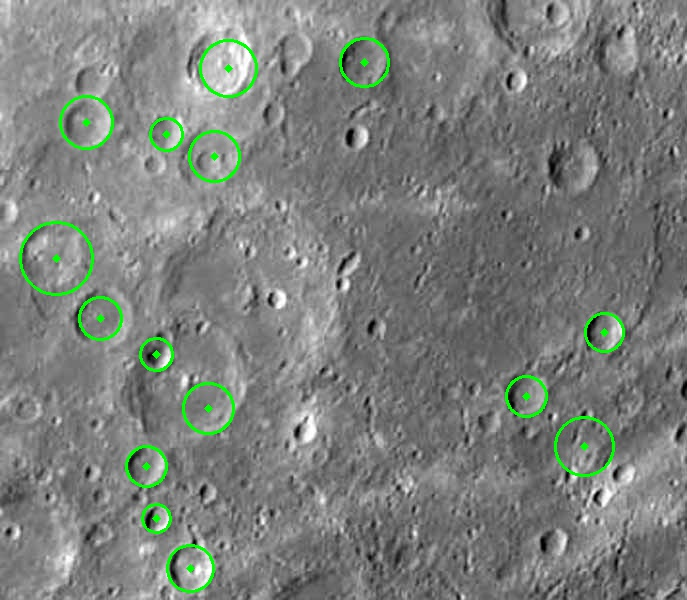## Deep Learning

Instead of training a deep convolutional neural network from scratch, we decided to bootstrap a neural network using the TensorFlow Object Detection API and images from NASA. The base model we trained on was originally trained on the COCO dataset with the architecture of award winning Microsoft’s 152 layer residual neural network. We trained the model for nearly 10 hours and got these results at the end.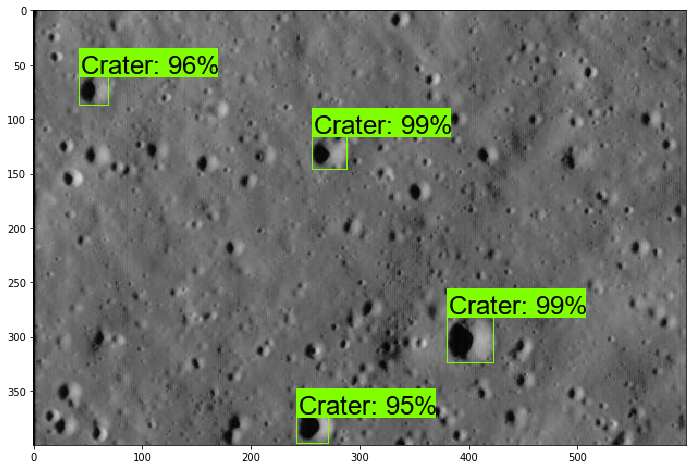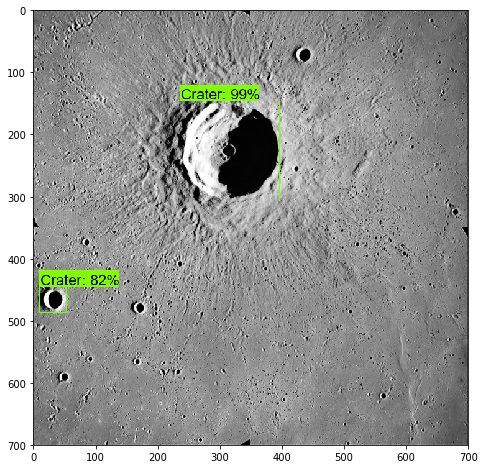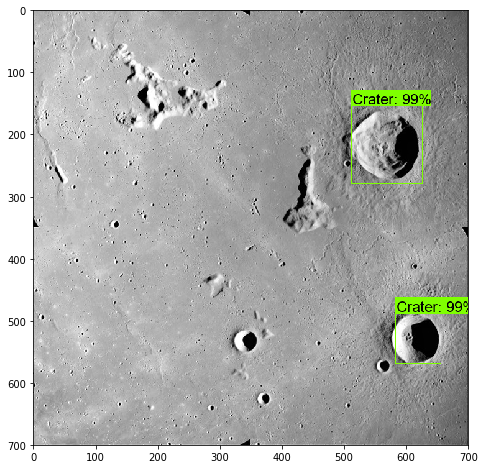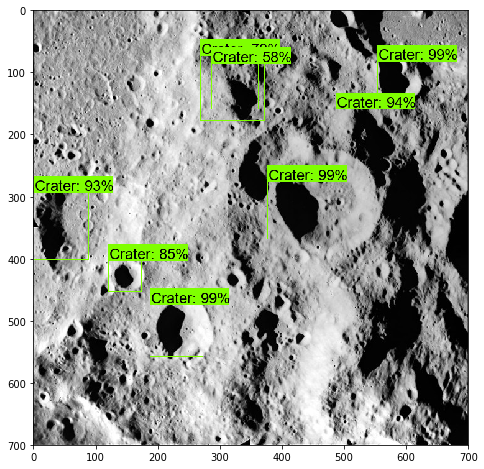## Tracking

Note: This goes into more detail than the report

Tracking craters presented a problem. We began by comparing the cropped images of craters against one another by computing the norm of the difference of pixels. But, we are not making using of another import result we are calculating. The rate at which a crater moves across the camera depends on the speed of the satellite. And under normal conditions, the motion of the crater can be seen as continuous. So, if the distance between the center of two craters across two consecutive frames is small, then it is likely that the two craters are the same.

Thus, we have two different parameters - one that tries to say that two craters are different (the norm of difference), and another that tries to say that two craters are the same (the euclidean distance between the centers). With this, we can construct a new cost function as follows:

$Cost(X, Y)= \alpha\frac{\left\lVert {X − Y} \right\rVert}{\left\lVert {X^{0} − Y} \right\rVert} + \beta(\left\lVert {X_c − Y_c} \right\rVert - 0.5)$

Here $$\alpha$$ is a hyperparameter that we can tune. We also normalize the norm of the difference to keep the errors within a small range. $$X^{0}$$ is the first crater against which we compare $$Y$$. So, the first term becomes just $$\alpha$$ when we compare agains the first image $$X^{0}$$. $$\beta$$ is a parameter that depends on the rate at which craters move across the camera i.e., the speed of the satellite. In fact, we can easily see that $$\beta$$ depends inversely on the speed. $$X_c$$ and $$Y_c$$ are the vectors representing the centers of the craters $$X$$ and $$Y$$ respectively.

Now, we can generalize an say that two craters are different if their cost is greater than a threshold we set, say $$\lambda$$. Otherwise, we assign the crater a new tag. Here are some results we got using our algorithm.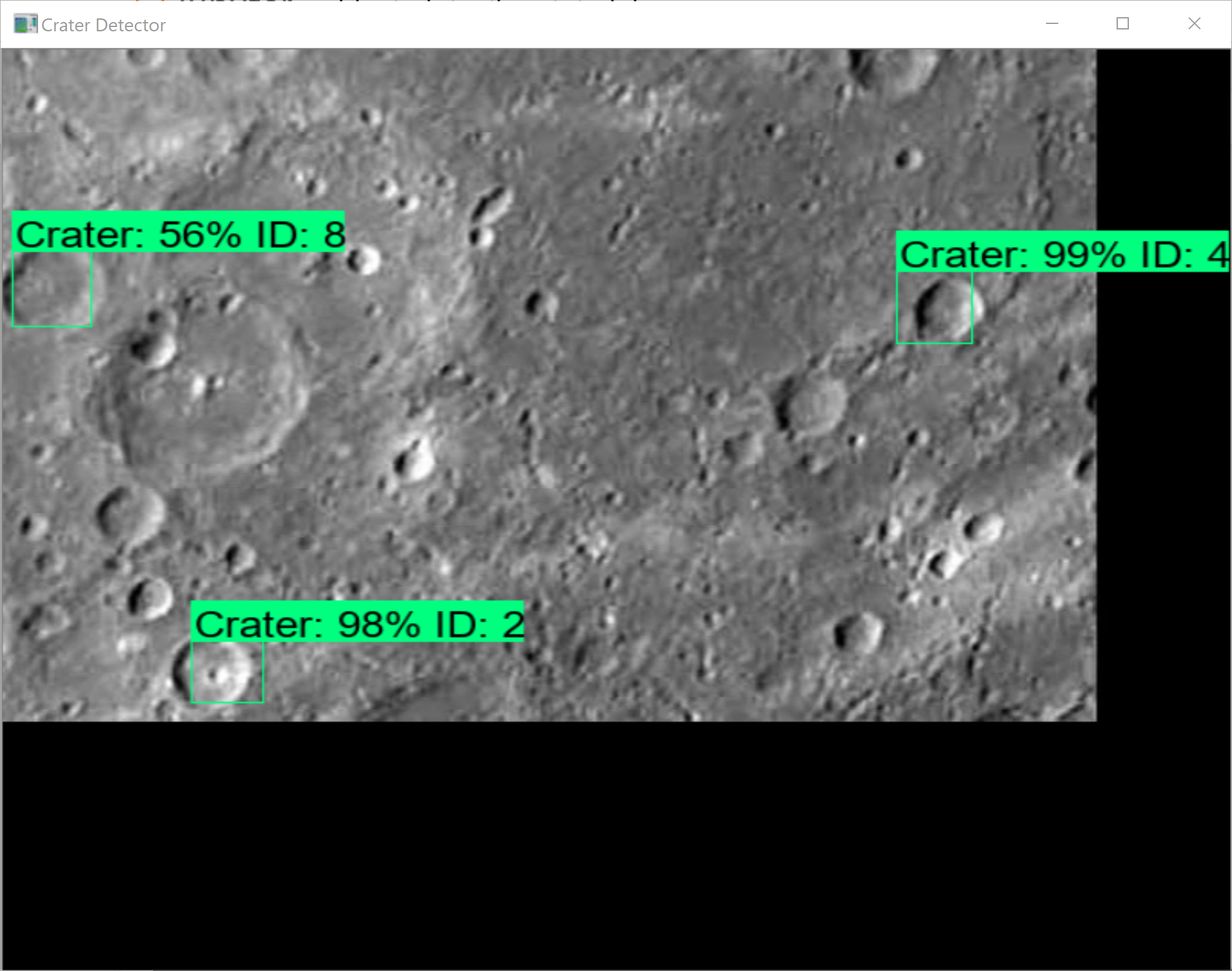## Conclusion

On the whole, we can conclude that traditional image processing techniques are not consistent. They need lots of preprocessing and manual fine tuning to work, which is what we are trying to avoid. Neural networks yield much better results and are also efficient – takes ~0.4s for a single 600x400px image.

Our preliminary tracking is sometimes lacking in robustness. Sometimes, we observed that the same crater gets different tags, and different craters get the same tag. We need to implement a third feature that penalizes the algorithm (increases the cost) if the crater has not been seen for a while. We could also try incorporating a simple Kalman filter that predicts the position of craters to assist our algorithm.

## Future Research

• We need to be able to track craters consistently, reliably, and efficiently. We need to improve upon our preliminary algorithm and increase the accuracy of our algorithm.
• We need to start modelling our algorithms under different lighting conditions and angles, which is more realistic.
• We need to use our results as inputs to navigation filters such as the Kalman Filter.

Clearly, we have a long way to go before this can be put to practice. But, this is a step in the right direction.

## Acknowledgements

 Simonyan, Zisserman (2014) “Very Deep Convolutional Neural Networks for Large-Scale Image Recognition

 Szegedy, Liu et. al (2015) “GoogLeNet

 Girshick, Donahue, et. al. (2014) “Rich feature hierarchies for accurate object detection and semantic segmentation

 Urbach, Stepinski (2009) “Automatic detection of sub-km craters in high resolution planetary images

 Kalal, Mikolajczyk, Matas (2010) “Tracking-Learning-Detection

 Dor, Tsiotras “Application of ORB-SLAM to Spacecraft Non-Cooperative Rendezvous

 Mur-Artal, Tardos (2016) “ORB-SLAM2: An Open-Source SLAM System for Monocular, Stereo, and RGB-D Cameras

 Ross Girshick (2015) “Fast R-CNN

Datasets

 NASA Detecting Crater Impact Challenge - https://www.nasa.gov/feature/detecting-crater-impact-challenge

 COCO Dataset - http://cocodataset.org

Tools

 TensorFlow Object Detection API - https://github.com/tensorflow/models/tree/master/research/object_detection

 OpenCV - https://opencv.org